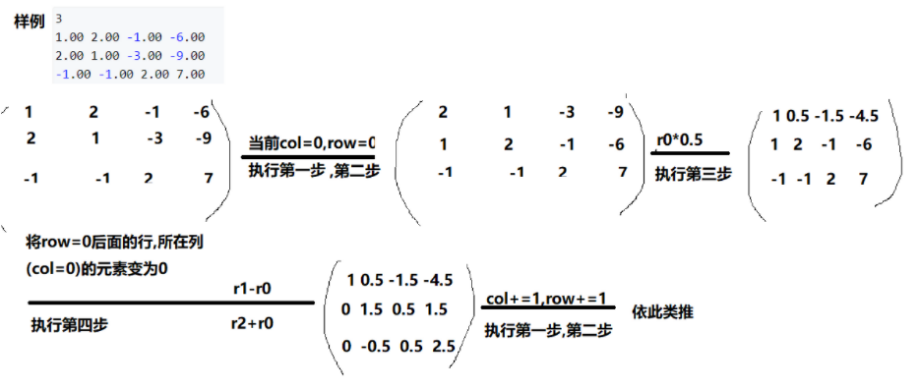# [数论](6)高斯消元

C++ 初等行列变化: 1.某一行乘上一个非零数,矩阵不变 2.某一行乘上一个常数加到另一行上,矩阵不变 3.交换矩阵中某两行的元素

思路:利用初等行列变化初等行列变化将矩阵变换成上三角形矩阵,再由后往前推出解



C++

1.筛选出所在列元素最大的行
2.将步骤1筛选出的行与所在行进行交换
3.将所在行所在列元素变为1
4.将所在行所在列的后面行的所在列元素变为0C++ const int N = 110; const double eps = 1e-6; int n; double a[N][N]; int gauss() { int c, r;// c 代表 列 col ， r 代表 行 row for (c = 0, r = 0; c < n; c ++ ) { int t = r;// 先找到当前这一列，绝对值最大的一个数字所在的行号 for (int i = r; i < n; i ++ ) if (fabs(a[i][c]) > fabs(a[t][c])) t = i;

    if (fabs(a[t][c]) < eps) continue;// 如果当前这一列的最大数都是 0 ，那么所有数都是 0，就没必要去算了，因为它的约束方程，可能在上面几行

for (int i = c; i < n + 1; i ++ ) swap(a[t][i], a[r][i]);//// 把当前这一行，换到最上面（不是第一行，是第 r 行）去
for (int i = n; i >= c; i -- ) a[r][i] /= a[r][c];// 把当前这一行的第一个数，变成 1， 方程两边同时除以 第一个数，必须要到着算，不然第一个数直接变1，系数就被篡改，后面的数字没法算
for (int i = r + 1; i < n; i ++ )// 把当前列下面的所有数，全部消成 0
if (fabs(a[i][c]) > eps)// 如果非0 再操作，已经是 0就没必要操作了
for (int j = n; j >= c; j -- )// 从后往前，当前行的每个数字，都减去对应列 * 行首非0的数字，这样就能保证第一个数字是 a[i] -= 1*a[i];
a[i][j] -= a[r][j] * a[i][c];

r ++ ;// 这一行的工作做完，换下一行
}

if (r < n)// 说明剩下方程的个数是小于 n 的，说明不是唯一解，判断是无解还是无穷多解
{// 因为已经是阶梯型，所以 r ~ n-1 的值应该都为 0
for (int i = r; i < n; i ++ )//
if (fabs(a[i][n]) > eps)// a[i][n] 代表 b_i ,即 左边=0，右边=b_i,0 != b_i, 所以无解。
return 2;
return 1;// 否则， 0 = 0，就是r ~ n-1的方程都是多余方程
}
// 唯一解 ↓，从下往上回代，得到方程的解
for (int i = n - 1; i >= 0; i -- )
for (int j = i + 1; j < n; j ++ )
a[i][n] -= a[j][n] * a[i][j];//因为只要得到解，所以只用对 b_i 进行操作，中间的值，可以不用操作，因为不用输出

return 0;`

}

## 本文链接：

https://nullcode.fun/144.html
1 + 6 =